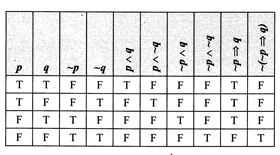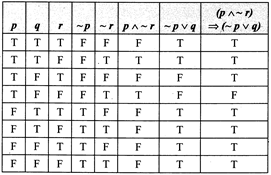# JEE Main Mathematical Reasoning Previous Year Questions With Solutions

Mathematical reasoning deal to determine the truth values of the given statements. This topic is covered under the JEE Main only and not in JEE Advanced. It carries four marks and is one of the easiest concepts in the entire syllabus. Mathematical Logic is a subject which deals with the principles of reasoning. Mathematical reasoning is also called a science of proof. In this article, JEE aspirants can get a set of questions asked in previous year exams on Mathematical reasoning with detailed solutions.

## JEE Main Mathematical Reasoning Previous Year Questions With Solutions

Question 1: The contrapositive of the inverse of p ⇒ ~q is

Solution:

The inverse of p ⇒ ∼q is ∼ p ⇒ q

The contrapositive of ∼ p ⇒ q is ∼ q ⇒ p. [Because the contrapositive of p ⇒ q is ∼ q ⇒ p.]

Question 2: Which of the following is the contrapositive of if two triangles are identical, then these are similar??

A) if two triangles are not similar, they are not identical

B) If two triangles are not identical, then these are not similar

C) If two triangles are not identical, then these are similar

D) If two triangles are not similar, then these are identical

Solution:

Consider the following statements

p: Two triangles are identical.

q: Two triangles are similar.

Clearly, the given statement in symbolic form is p ⇒ q.

Therefore, its contrapositive is given by ∼ q ⇒ ∼ p

Now,

∼p: two triangles are not identical.

∼q: two triangles are not similar.

Therefore, ~ q ⇒ ~ p: If two triangles are not similar, then these are not identical.

Question 3: If p is true and q is false, then which of the following statements is not true?

A) p ∨ q

B) p ⇒ q

C) p ∧ ( ~q)

D) p ⇒ p

Solution:

When p is true and q is false, then p∨q is true, q ⇒ p is true and p ∧ (∼q) is true. (Therefore, both p and ∼q are true)

Here, p ⇒ q is not true as a true statement cannot imply a false statement.

Question 4: ~( p ∨ q) ∨ (~p ∧ q) is equivalent to ____________.

Solution:

∼ (p ∨ q) ∨ (∼p ∧ q) = (∼p ∧ ∼q) ∨ (∼p ∧ q) =∼q ∧ ∼(∼ (p ∧ q))

Question 5: Which of the following is logically equivalent to ~( ~ p ⇒ q)?

A) p ∧ q

B) p ∧ ~q

C) ~p ∧ q

D) ~p ∧ ~q

Solution:

It is clear from the table that ∼ (∼p ⇒ q) is equivalent to ∼p ∧ ∼q.Question 6: If (p ∧ ~r) ∧ ( ~p / q) is false, then write the truth values of p, q and r.

Solution:Since,

(p ∧ ∼r) ⇒ (∼p ∨ q) is F Then, p = T, q = F, r = F.

Question 7: If p and q are two statements, then (p ⇒ q) ⇔ ( ~q ⇒ ~p) is a ___________.

Solution:

 p⇒q ∼p⇒∼q p⇒q⇔∼q⇒∼p T T T F F T T T T T T T

Therefore, it is a tautology. Hence, the given proposition is a tautology.

Question 8: If each of the following statements is true, then P ⇒ ~q, q ⇒ r, ~r

A) p is false

B) p is true

C) q is true

D) None of these

Solution:

Since ∼r is true, therefore, r is false. Also, q ⇒ r is true, therefore, q is false. (Therefore, a true statement cannot imply a false one) Also, p ⇒ q is true, therefore, p must be false.

Question 9: What is the negation of the compound proposition?

If the examination is difficult, then I shall pass if I study hard.

Solution:

If p: Examination is difficult

q: I shall pass

r: I study hard

Given result is P ⇒ (r ⇒ q)

Now, ∼ (r ⇒ q) = r ∧ ∼q ∼(p ⇒ (r ⇒ q)) = p ∧ (r ∧ ∼q)

The examination is difficult and I study hard but I shall not pass.

Question 10: The statement p → (p → q) is equivalent to __________.

Solution:

p → (q → p) = −p (q → p) = ∼p ∨ (∼q ∨ p)

(Since p ∨∼p is always true) = ∼p ∨ p ∨ q = p → (p ∨ q)

Question 11: The statement ~(p ↔ ~q)is ________.

Solution:

 p q ∼q p↔∼q ∼(p↔∼q) p↔q T T F F T T T F T T F F F T F T F F F F T F T T

Question 12: The Boolean Expression (p ∧ ~q) ∨ q ∨( ~p ∧ q) is equivalent to ___________.

Solution:

[(p ∧ ∼q) ∨ q] ∨ (∼p ∧ q) = (p ∨ q) ∧ (∼q ∨ q) ∨ (∼p ∧ q)

= (p ∨ q) ∧ [t ∨ (∼p ∧ q)]

= (p ∨ q) ∧ t

= p ∨ q

Question 13:

 Consider the following statements P: Suman is brilliant Q: Suman is rich R: Suman is honest

The negation of the statement: Suman is brilliant and dishonest if and only if Suman is rich?? can be expressed as

Solution:

Suman is brilliant and dishonest is P∧∼R.

Suman is brilliant and dishonest if and only if Suman is rich is Q ↔ (P ∧ ∼R)

Negative of the statement is expressed as ∼(Q ↔ (P ∧ ∼R).